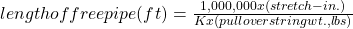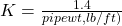# Free Point Calculation For Stuck Pipe

When a pipe becomes stuck in the wellbore for any reason, one of the first steps is to calculate to determine at what depth or point the pipe stuck has occurred. This process is called stuck pipe free point calculation. When retrieving production tubing from a well, it is often expected to find that the tubing is stuck, with the sealed unit seized, or locked, up in the production packer bore due to scale or an abundance of solids that have settled around the outside of the sealed unit in the tubing- casing annulus.

## Drill Pipe or Tubing Stretch

When tension is applied to a drill string, it elongates the drill string. Stretch in a drill pipe can be measured, and a calculation made to estimate the depth to the top of the stuck pipe. All pipe is elastic and all formulae and charts are based on the modulus of elasticity of steel, which is approximately 30,000,000 lb/sq.in. If the length of stretch in the pipe with a given pull (check Margin Of Overpull Drilling Calculations) is measured, the free pipe can be calculated or determined from a chart available in data books.

## Procedures for Determining Free Point in Stuck Pipe

Since all wellbores are crooked, friction exists between the pipe and the wellbore (Check Torque and drag in drilling). Steps should be taken to reduce this friction to a minimum. The pipe should be worked for a period of time by pulling approximately 10%-15% more than the weight of the string and then slacking off an equal amount. Some techniques reduce errors in estimating stuck points from stretch data. It is also necessary to assume certain arbitrary conditions. Stretch charts and formulas do not consider drill collars or heavy-weight drill pipe.

1. First, pull tension on the pipe at least equal to the average Top Drive hook load (air weight) of the pipe before getting stuck. This should then be marked on the pipe as point “a”.
2. Next, pull additional tension predetermined within the range of safe tensional limits on the pipe.
3. Now slack off this weight back down to the hook load weight. Mark this point “b”. It will be lower than point “a”. This difference is accounted for by friction of the pipe in the wellbore.
4. The next step in free point calculation for stuck pipe is to pull additional tension on the pipe to a predetermined amount within the safe working limits of the string. Mark this point as “c”.
5. Then pull additional tension on the pipe in the same amount used to determine points “a” and “b” and slack off to tension used to locate point “c”. Mark this point “d”.
6. The mid-point between “a” and “b” and between “c” and “d” will be the marks used.
7. Measure the distance between these average marks and use this number as the stretch in inches. The amount of free pipe can be determined by using the following formula:Where:

K = constant

The constant in this free point calculation formula for stuck pipe can be determined by:for drill pipefor Tubing and Casing

## Free Point Calculation For Stuck Pipe Review

This method of estimating the stuck point of pipe is not completely reliable and accurate as there are many variables caused by friction (check also: Free point indicator), doglegs (Dogleg Severity), hole angle, and pipe wear. However, it frequently indicates the cause of sticking such as possible areas of a key seat or differential sticking in open holes and collapsed tubing or tubing leaks in producing wells.

In addition to the basic formula provided above for calculating the amount of free pipe, there are reference manuals available that provide stretch charts from which the length of free pipe can be read directly. The same procedures and precautions, as outlined above, should be followed to obtain the pipe stretch with a predetermined pull over the string weight.

The accuracy of the charts and the formula is approximately the same, as both are affected by the same problems of hole friction, loss of material in used pipe, and the accuracy of weight indicators. Note, however, that the modulus of elasticity of all grades of steel is the same. The grade of the pipe does not affect its stretch. Also, when pipe is stuck, buoyancy forces are not effective. Immediately when the pipe is freed, the buoyant forces are again in effect and should be considered accordingly.

## Example

13,000 feet of 23 #/ft casing is stuck near the bottom of the well. A differential load of 55,000 lb was applied on the stuck pipe which resulted in a stretch of 42in. Calculate what is the depth of the stuck pipe free point?

Solution

K = 1.4 / 23 = 0.0608

Pull = 55000 lb

Stretch = 42 in

Length of free pipe = 1000000 x 42 / 0.0608 x 55000= 12559 ft